# JP5105201B2 - Angle detection apparatus and angle detection method - Google Patents

## Info

Publication number
JP5105201B2
JP5105201B2 JP2009030093A JP2009030093A JP5105201B2 JP 5105201 B2 JP5105201 B2 JP 5105201B2 JP 2009030093 A JP2009030093 A JP 2009030093A JP 2009030093 A JP2009030093 A JP 2009030093A JP 5105201 B2 JP5105201 B2 JP 5105201B2
Authority
JP
Japan
Prior art keywords
rotating
angle
rotation
magnetic
detection
Prior art date
Legal status (The legal status is an assumption and is not a legal conclusion. Google has not performed a legal analysis and makes no representation as to the accuracy of the status listed.)
Active
Application number
JP2009030093A
Other languages
Japanese (ja)
Other versions
JP2010054495A (en
Inventor

Original Assignee
Ｔｄｋ株式会社
Priority date (The priority date is an assumption and is not a legal conclusion. Google has not performed a legal analysis and makes no representation as to the accuracy of the date listed.)
Filing date
Publication date
Priority to JP2008196456 priority Critical
Priority to JP2008196456 priority
Application filed by Ｔｄｋ株式会社 filed Critical Ｔｄｋ株式会社
Priority to JP2009030093A priority patent/JP5105201B2/en
Publication of JP2010054495A publication Critical patent/JP2010054495A/en
Application granted granted Critical
Publication of JP5105201B2 publication Critical patent/JP5105201B2/en
Active legal-status Critical Current
Anticipated expiration legal-status Critical

• 238000001514 detection method Methods 0.000 title claims description 20
• 230000005291 magnetic Effects 0.000 claims description 201
• 230000000875 corresponding Effects 0.000 claims description 30
• 238000006243 chemical reactions Methods 0.000 claims description 25
• 238000004364 calculation methods Methods 0.000 claims description 23
• 230000000694 effects Effects 0.000 claims description 23
• 235000020127 ayran Nutrition 0.000 claims description 17
• 239000010410 layers Substances 0.000 claims description 16
• 230000000295 complement Effects 0.000 claims description 4
• 239000003302 ferromagnetic materials Substances 0.000 claims description 4
• 230000005389 magnetism Effects 0.000 claims description 2
• 238000005070 sampling Methods 0.000 description 12
• 230000005415 magnetization Effects 0.000 description 11
• 230000004907 flux Effects 0.000 description 7
• 230000014509 gene expression Effects 0.000 description 6
• 238000010586 diagrams Methods 0.000 description 3
• 238000006073 displacement reactions Methods 0.000 description 3
• 239000000203 mixtures Substances 0.000 description 2
• 239000007787 solids Substances 0.000 description 2
• 239000003570 air Substances 0.000 description 1
• 229910052803 cobalt Inorganic materials 0.000 description 1
• 239000010941 cobalt Substances 0.000 description 1
• 229910052742 iron Inorganic materials 0.000 description 1
• 239000011514 iron Substances 0.000 description 1
• 238000000034 methods Methods 0.000 description 1
• 229910052759 nickel Inorganic materials 0.000 description 1
• 239000010950 nickel Substances 0.000 description 1
• 238000010248 power generation Methods 0.000 description 1
• 230000001629 suppression Effects 0.000 description 1

## Images

•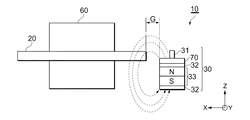•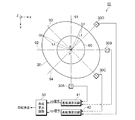•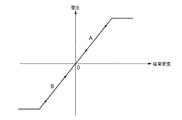•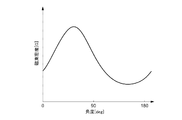•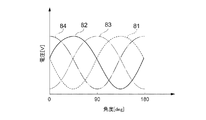•••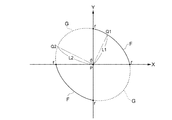•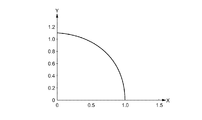•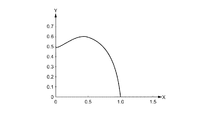•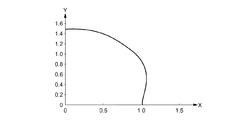•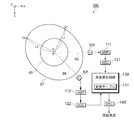•••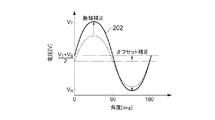•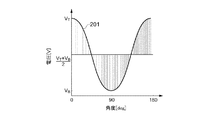•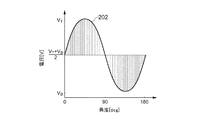••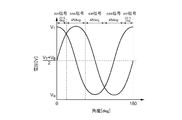•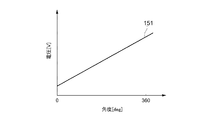## Classifications

• GPHYSICS
• G01MEASURING; TESTING
• G01BMEASURING LENGTH, THICKNESS OR SIMILAR LINEAR DIMENSIONS; MEASURING ANGLES; MEASURING AREAS; MEASURING IRREGULARITIES OF SURFACES OR CONTOURS
• G01B7/00Measuring arrangements characterised by the use of electric or magnetic means
• G01B7/30Measuring arrangements characterised by the use of electric or magnetic means for measuring angles or tapers; for testing the alignment of axes

## Description

The present invention relates to an angle detection device and an angle detection method for calculating a rotation angle of a rotation shaft.

Japanese Patent Application Laid-Open No. 2001-91208 discloses a magnetic detecting element that generates a magnetic field between a magnet and a yoke as a means for detecting a rotation angle of a detection object such as a throttle valve and is disposed in the magnetic field. There has been proposed a rotation angle detection device having a configuration in which the position of the rotation is shifted from the rotation center of the rotor. When the position of the magnetic detection element is shifted from the rotation center of the rotor, the relationship between the magnetic flux angle linked to the magnetic detection element and the rotation angle of the rotor changes. By utilizing this change, it becomes possible to arbitrarily set the output characteristic of the magnetic detection element with respect to the rotation angle in a wide range, and the detection characteristic of the rotation angle can be improved.

JP 2001-91208 A

However, the above-described rotation angle detection device has a difficulty in that there is a certain limitation on the detection angle (see FIG. 8B).

Therefore, an object of the present invention is to propose an angle detection device and an angle detection method in which the detection angle is not limited.

In order to solve the above problems, an angle detection device according to the present invention is a rotating body fixed to a rotating shaft, and defines an XY orthogonal coordinate system on a rotating plane of the rotating body with the rotation center of the rotating body as a coordinate origin. Then, for any integer n greater than or equal to 2, the distance between each of the two points at which two straight lines intersecting the rotation center at the intersection angle of (π / n) intersect the outer periphery of the rotating body and the rotation center And the plane shape obtained by projecting the rotator onto the plane of rotation is symmetric with respect to Y = tan ((π / 2n)) X, and (π / n) with respect to the center of rotation. First and second magnetic sensors arranged near the outer periphery of the rotating body with an angular difference, the first and second magnetic sensors between the outer periphery of the rotating body and the first magnetic sensor periodically changing as the rotating body rotates Detects a change in the magnetic field corresponding to a change in the distance, and outputs a first detection signal. The first magnetic sensor detects a change in the magnetic field corresponding to a change in the second distance between the outer circumference of the rotating body and the second magnetic sensor that changes in a complementary manner following the change in the first distance. A second magnetic sensor for outputting a second detection signal, a differential calculation means for differentially calculating the first detection signal and the second detection signal and outputting a differential signal, and a differential signal Angle calculation means for calculating the rotation angle of the rotation shaft based on the rotation angle.

According to the angle detection device of the present invention, the two straight lines intersecting the rotation center of the rotating body at an intersection angle of (π / n) intersect each of the two points where the outer periphery of the rotating body intersects the rotation center of the rotating body. A rotating body is used in which the sum of the respective distances between them is constant and the planar shape obtained by projecting the rotating body onto the rotation plane is symmetric with respect to Y = tan ((π / 2n)) X. Differential calculation of the first and second detection signals output from each of the first and second magnetic sensors disposed near the outer periphery of the rotating body with an angular difference of (π / n) with respect to the rotation center The differential signal obtained by doing so becomes a substantially sine wave signal including the rotation angle information of the rotating body, and angle detection with a small detection error can be performed over the entire angle (0 degrees to 360 degrees) range. Further, since the rotating body does not have to be attached to the end face of the rotating shaft, the angle detecting device is easily attached.

Here, it is desirable that the distance between the first magnetic sensor and the rotation center of the rotating body is equal to the distance between the second magnetic sensor and the rotation center of the rotating body. . According to such a configuration, since the first and second detection signals output from the first and second magnetic sensors have sufficient symmetry, the differential signal can be brought close to an ideal sine wave. it can.

Further, it is desirable that the shape obtained by projecting the rotating body on the plane of rotation has a shape in which 2n partial ellipses having a central angle (π / n) are combined. According to such a shape, the first and second magnetic sensors output from the first and second magnetic sensors arranged near the outer periphery of the rotating body with an angular difference of (π / n) with respect to the rotation center of the rotating body. Since the second detection signal is an approximately sine wave signal for n periods that takes n maximum values and n minimum values when the rotating body makes one revolution, the first and second detection signals are The differential signal obtained by performing the differential operation is a substantially sine wave signal for n cycles that is very similar to an ideal sine waveform including the rotation angle information of the rotating body. In addition, as for the shape which projected the rotary body on the rotation plane, what does not have a trough part is still more desirable. This is because the magnetic field is likely to be disturbed in the valley.

In a preferred embodiment of the present invention, the rotating body is made of a ferromagnetic material. The first magnetic sensor includes a first magnet that generates a magnetic field between the outer periphery of the rotating body and the first magnetic sensor, and a first detection based on a magnetic field that changes according to a change in the first distance. A first magnetoresistive element that outputs a signal. The second magnetic sensor includes a second magnet that generates a magnetic field between the outer periphery of the rotating body and the second magnetic sensor, and a second detection in accordance with the magnetic field that changes in accordance with a change in the second distance. A second magnetoresistive element that outputs a signal. According to such a configuration, with the rotation of the rotating body, the first distance between the rotating body and the first magnetic sensor and the second distance between the rotating body and the second magnetic sensor are Since these changes appear as changes in the resistance values of the first and second magnetic sensors, the first and second detection signals include information on the rotation angle of the rotating body.

In a preferred embodiment of the present invention, the first magnetoresistive element includes a first free magnetic layer whose longitudinal direction is set in the direction of the rotation center of the rotating body. The second magnetoresistive element includes a second free magnetic layer whose longitudinal direction is set in the direction of the rotation center of the rotating body. By setting the longitudinal direction of the free magnetic layer to the rotation center direction, the angle detection accuracy can be increased.

The angle detection method according to the present invention is arranged in the vicinity of the outer periphery of the rotating body with an angle difference of (π / n) with respect to an arbitrary integer n of 2 or more with respect to the rotation center of the rotating body fixed to the rotating shaft. A method for detecting a rotation angle of a rotation axis using a first and a second magnetic sensor, wherein an XY orthogonal coordinate system is defined on a rotation plane of a rotating body with the rotation center as a coordinate origin and (π / N), the sum of the distances between each of the two points intersecting the outer periphery of the rotating body and the rotation center is constant, and the rotating body is projected onto the rotating plane. Between the step of rotating the rotating body whose plane shape is symmetric with respect to Y = tan ((π / 2n)) X, and the outer periphery of the rotating body periodically changing with the rotation of the rotating body and the first magnetic sensor Detecting the first magnetic field change corresponding to the first distance change A step of outputting a first detection signal from the air sensor, and a change in the second distance between the outer periphery of the rotating body and the second magnetic sensor that change complementarily in accordance with the change in the first distance. Outputting a second detection signal from a second magnetic sensor that detects a corresponding magnetic field change; and outputting a differential signal by performing a differential operation on the first detection signal and the second detection signal; And calculating a rotation angle of the rotating shaft based on the differential signal.

According to the angle detection method of the present invention, each of two points where two straight lines intersecting the rotation center of the rotating body at an intersection angle of (π / n) intersect the outer periphery of the rotating body and the rotation center of the rotating body. A rotating body is used in which the sum of the respective distances between them is constant and the planar shape obtained by projecting the rotating body onto the rotation plane is symmetric with respect to Y = tan ((π / 2n)) X. Differential calculation of the first and second detection signals output from each of the first and second magnetic sensors disposed near the outer periphery of the rotating body with an angular difference of (π / n) with respect to the rotation center The differential signal obtained by doing so becomes a substantially sine wave signal including the rotation angle information of the rotating body, and angle detection with a small detection error can be performed over the entire angle (0 degrees to 360 degrees) range.

An angle detection device according to another aspect of the present invention is a rotating body fixed to a rotating shaft, and when an XY orthogonal coordinate system is defined on a rotating plane of the rotating body with the rotation center of the rotating body as a coordinate origin, 2 For the above-mentioned arbitrary integer n, the sum of the distances between the two points where the two straight lines intersecting the rotation center at the intersection angle of (π / n) and the outer periphery of the rotating body and the rotation center is constant. And a rotating body whose plane shape which is projected onto the rotating plane is symmetric with respect to Y = tan ((π / 2n)) X, and rotated by an angular difference of (π / 2n) with respect to the center of rotation. A first and a second magnetic sensor arranged near the outer periphery of the body, wherein the first distance between the outer periphery of the rotating body and the first magnetic sensor changes periodically with the rotation of the rotating body. A first magnetic sensor that detects a magnetic field change corresponding to the change and outputs a first detection signal. And detecting a magnetic field change corresponding to a change in the second distance between the outer circumference of the rotating body and the second magnetic sensor, which periodically changes as the rotating body rotates, and outputs a second detection signal The second magnetic sensor, the conversion table storing the rotation angle of the rotating shaft corresponding to the first and second detection signals, and the first and second detections output from the first and second magnetic sensors Angle calculating means for comparing the signal and the conversion table and outputting the rotation angle of the rotating body is provided.

According to the angle detection apparatus of the present invention, when an XY orthogonal coordinate system is defined on the rotation plane of the rotating body with the rotation center of the rotating body as the coordinate origin, an arbitrary integer n of 2 or more (π / n is a plane in which the sum of the distances between the two points intersecting the outer circumference of the rotating body and the rotation center is constant, and the rotating body is projected onto the rotating plane. Since a rotating body whose shape is symmetric with respect to Y = tan ((π / 2n)) X is used, a phase difference (mechanical angle) of (π / 2n) with respect to the rotation center of the rotation body and from the rotation center. The distance between the first and second magnetic sensors arranged at equal distances and the outer periphery of the rotating body changes periodically. Since the first and second detection signals output from the first and second magnetic sensors include information on the rotation angle of the rotating body and have a phase difference (electrical angle) of 90 deg from each other, By performing functional processing on the first and second detection signals, the rotation angle of the rotating body can be obtained.

In a preferred embodiment of the present invention, the first and second detection signals are substantially sinusoidal signals, and the angle calculation means is an angle at which the detection signal takes an intermediate value among the first and second detection signals. On the other hand, it is preferable to output the rotation angle of the rotating body by comparing the detection signal within the angle range of ± 22.5 deg with the conversion table. By reading a value near an intermediate value with a large amplitude change amount rather than a value near a peak of a signal waveform with a small amplitude change amount, the influence on noise can be suppressed.

According to another aspect of the present invention, there is provided an angle detection method in the vicinity of the outer periphery of a rotating body with an angle difference of (π / 2n) with respect to an arbitrary integer n of 2 or more with respect to the rotation center of the rotating body fixed to the rotating shaft The rotation angle of the rotation axis is detected using the first and second magnetic sensors arranged on the rotation axis when the XY orthogonal coordinate system is defined on the rotation plane of the rotating body with the rotation center as the coordinate origin. The sum of the distances between each of the two points where the two straight lines intersecting at the center at an intersection angle of (π / n) intersect the outer periphery of the rotating body and the rotation center is constant, and the rotating body is rotated. A step of rotating a rotating body whose plane shape projected onto the plane is symmetric with respect to Y = tan ((π / 2n)) X, an outer periphery of the rotating body that periodically changes with the rotation of the rotating body, and the first magnetism Detect magnetic field changes corresponding to changes in the first distance to the sensor. A step of outputting a first detection signal from the first magnetic sensor, and a change in the second distance between the outer periphery of the rotating body and the second magnetic sensor that periodically changes as the rotating body rotates. A step of outputting a second detection signal from the second magnetic sensor for detecting a magnetic field change corresponding to the first, a conversion table for storing the rotation angle of the rotation shaft corresponding to the first and second detection signals, Comparing the first and second detection signals output from the first and second magnetic sensors and outputting the rotation angle of the rotating body.

According to the angle detection method of the present invention, when the XY orthogonal coordinate system is defined on the rotation plane of the rotator with the rotation center of the rotator as the coordinate origin, an arbitrary integer n of 2 or more (π / n is a plane in which the sum of the distances between the two points intersecting the outer circumference of the rotating body and the rotation center is constant, and the rotating body is projected onto the rotating plane. Since a rotating body whose shape is symmetric with respect to Y = tan ((π / 2n)) X is used, a phase difference (mechanical angle) of (π / 2n) with respect to the rotation center of the rotation body and from the rotation center. The distance between the first and second magnetic sensors arranged at equal distances and the outer periphery of the rotating body changes periodically. Since the first and second detection signals output from the first and second magnetic sensors include information on the rotation angle of the rotating body and have a phase difference (electrical angle) of 90 deg from each other, By performing functional processing on the first and second detection signals, the rotation angle of the rotating body can be obtained.

According to the present invention, it is possible to provide an angle detection device and an angle detection method in which the detection angle is not limited.

It is explanatory drawing which shows schematic structure of the angle detection apparatus concerning this embodiment. It is explanatory drawing which shows the system configuration | structure of the angle detection apparatus concerning this embodiment. It is a graph which shows the output characteristic of a magnetoresistive effect element. It is a graph which shows the change of the magnetic flux density with respect to the rotation angle of a rotary body. It is a graph which shows two detection signals output from a pair of magnetic sensors. It is a graph which shows the differential signal obtained by carrying out differential operation of the two detection signals output from a pair of magnetic sensor. It is explanatory drawing which shows the calculation method of the planar shape of a rotary body. It is explanatory drawing which shows the calculation method of the planar shape of a rotary body. It is a graph of elliptic function H (X, Y) when a = 0.9. It is a graph of elliptic function H (X, Y) when a = 1.5. It is a graph of elliptic function H (X, Y) when a = a = 0.5. It is explanatory drawing which shows schematic structure of the angle detection apparatus concerning Example 2. FIG. It is a graph which shows the cos signal and sin signal before calibration execution. It is explanatory drawing which shows the amplitude correction and offset correction of a cos signal. It is explanatory drawing which shows the amplitude correction and offset correction of a sin signal. It is explanatory drawing which shows the digital sampling of a cos signal. It is explanatory drawing which shows the digital sampling of a sin signal. It is explanatory drawing of a conversion table. It is explanatory drawing which shows the reading range of a cos signal and a sin signal. It is a graph of a linear output value.

Embodiments according to the present invention will be described below with reference to the drawings. In each embodiment, the same reference numerals indicate the same members, and duplicate descriptions are omitted.

FIG. 1 is an explanatory diagram showing a schematic configuration of an angle detection apparatus 10 according to the present embodiment.
The angle detection device 10 includes a rotating body (rotor) 20 fixed to the rotating shaft 60 and a magnetic sensor 30 disposed near the outer periphery of the rotating body 20 as main components. The rotating body 20 is a rotor made of a ferromagnetic material (for example, iron, cobalt, nickel, etc.). The rotation shaft 60 is a rotation shaft (for example, a vehicle drive shaft, a motor drive shaft, or the like) that rotates by receiving a driving force from a power generation source or the like, and its axial direction is the Z direction. When the rotating shaft 60 rotates, the rotating body 20 rotates in the XY plane.

The magnetic sensor 30 includes a magnet 33 that functions as a magnetic field generating means for generating an external magnetic field, and a magnetoresistive effect element 31 that detects a change in the external magnetic field as a voltage change. The magnetoresistive effect element 31 may have a half-bridge configuration or a full-bridge configuration. As a mounting form of the magnetic sensor 30, the magnetoresistive effect element 31 is arranged on the surface of the printed wiring board 70 so that the magnetoresistive effect element 31 is positioned on a straight line in the Z direction passing through the center point of the magnet 33. It is preferable to arrange the magnet 33 on the back surface of the magnet. Further, in order to efficiently collect an external magnetic field generated from the magnet 33, it is preferable to arrange the yokes 32 on both poles of the magnet 33.

The magnetoresistive element 31 has a magnetization direction set to a specific direction, and is configured such that the magnetization state (for example, the magnetization direction and the strength of magnetization) is not affected by the displacement of the external magnetic field. A magnetic layer (not shown) and a free magnetic layer (not shown) whose magnetization state is displaced by a change in the external magnetic field are provided. When the rotating body 20 rotates with the rotation of the rotating shaft 60, the gap G between the outer periphery of the rotating body 20 and the magnetoresistive effect element 31 changes periodically. When the gap G changes, the magnetic flux density drawn from the magnet 33 arranged on the back surface of the magnetoresistive effect element 31 through the magnetoresistive effect element 31 to the rotating body 20 changes. Then, since the magnetization state of the free magnetic layer in the magnetoresistive effect element 31 changes, the difference in magnetization state displacement between the magnetization state of the pinned magnetic layer where the magnetization state does not change and the free magnetic layer where the magnetization state changes. Will occur. This displacement difference in the magnetization state is a physical quantity that reflects the rotation angle of the rotating body 20, and specifically appears as a change in the resistance value of the magnetoresistive element 31. A bias current is supplied to the magnetoresistive effect element 31 from the printed wiring board 70, and a change in the resistance value of the magnetoresistive effect element 31 is detected as a change in the output voltage. The output voltage of the magnetoresistive effect element 31 is signal-processed as a detection signal indicating the rotation angle of the rotating body 20.

As shown in FIG. 3, the magnetoresistive effect element 31 operates in the region A or the region B where the relationship between the magnetic flux density passing through the magnetoresistive effect element 31 and the output voltage of the magnetoresistive effect element 31 is linear. Thus, it is preferable to design the strength of the external magnetic field, the average interval of the gap G, and the like. In this case, the output of the magnetic sensor has linearity according to the distance of the gap G. Further, the positional relationship in the thrust direction (axial direction) between the rotating body 20 and the magnetic sensor 30 is a position where the rotating body 20 does not deviate from the magnetoresistive effect element 31, including a shift due to a runout caused by the rotation of the rotating body 20. It is desirable to have a relationship. For example, when the mounting error is ± 0.5 mm, the runout is ± 0.5 mm, and the thickness of the magnetoresistive element 31 is 0.5 mm, the thickness of the rotating body 20 is desirably 3.0 mm or more.

FIG. 2 is an explanatory diagram showing a system configuration of the angle detection apparatus 10.
When the XY orthogonal coordinate system is defined on the rotation plane of the rotator 20 with the rotation center P of the rotator 20 as the coordinate origin, the shape of the rotator 20 projected onto the rotation plane (XY plane) (hereinafter referred to as a plane shape) Is the distance between each of the two points (Q1, Q2) where the two straight lines intersecting the rotation center P at the intersection angle φ = (π / 2) intersect the outer periphery of the rotating body 20 and the rotation center P ( L1 and L2) have a shape that satisfies the condition that the sum (L1 + L2) is constant and the plane shape of the rotating body 20 is symmetrical with respect to Y = X (where L1 is a line segment of the line segment PQ1) L2 is the line segment length of the line segment PQ2.) The planar shape of the rotating body 20 satisfying such a condition has a shape in which four partial ellipses having a central angle (π / 2) are combined. When the rotating body 20 makes one rotation, the planar shape is approximately two cycles. A sine wave detection signal is obtained. Considering the condition that the planar shape of the rotator 20 is symmetric with respect to Y = X and the condition that a detection signal for two periods is obtained when the rotator 20 makes one rotation, the ellipse of four partial ellipses is obtained. Note that there are two types of ratios in all.

It is desirable that the planar shape of the rotator 20 does not have a valley. This is because there is a high possibility that the magnetic field is disturbed in the valley, and it is not suitable for highly accurate angle detection.

A pair of magnetic sensors 30A and 30B are arranged in the vicinity of the outer periphery of the rotating body 20 with an angle difference of (π / 2) with respect to the rotation center P, and an angle of (π / 2) with respect to the rotation center P. Due to the difference, another pair of magnetic sensors 30C and 30D are arranged. A straight line 91 connecting the magnetic sensor 30A and the rotation center P is orthogonal to a straight line 92 connecting the magnetic sensor 30B and the rotation center P, and a straight line 93 connecting the magnetic sensor 30C and the rotation center P is the same as the magnetic sensor 30D. It is orthogonal to a straight line 94 connecting the rotation center P. The adjacent magnetic sensors 30A and 30C are arranged with an angle difference of (π / 4) with respect to the rotation center P, and the adjacent magnetic sensors 30B and 30D are (π / 4) with respect to the rotation center P. ). Thus, each magnetic sensor 30A, 30C, 30B, 30D is fixed at an angular difference of (π / 4) with respect to the rotation center P, and even if the rotating body 20 rotates, the rotation center P And the respective magnetic sensors 30A, 30C, 30B, 30D are always kept constant.

In order to distinguish the magnetic sensors 30A, 30B, 30C, and 30D shown in FIG. 2 from each other, different reference numerals are given for convenience, but substantially the same configuration as the magnetic sensor 30 shown in FIG. Have. For this reason, when it is not necessary to distinguish these magnetic sensors 30A, 30B, 30C, and 30D, they are simply referred to as the magnetic sensor 30.

Now, when the rotating body 20 is rotated halfway, as shown in FIG. 4, a change corresponding to one cycle appears in the waveform indicating the change in the magnetic flux density of the external magnetic field passing through the magnetic sensor 30. When the rotator 20 is divided in half by a line segment passing through the rotation center P, the half divide has a shape in which two partial ellipses having different central angles (π / 2) having different ellipse ratios are combined. Therefore, although the magnetic flux density waveform shown in FIG. 4 is not a sine waveform in a strict sense, the waveform is similar to the sine waveform. As described above, since the relationship between the magnetic flux density passing through the magnetoresistive effect element 31 and the output voltage of the magnetoresistive effect element 31 is linear, the detection signal output from the magnetic sensor 30 is similar to a sine wave. Has a waveform. The planar shape of the rotator 20 is between the rotation center P and each of two points (Q1, Q2) where two straight lines intersecting the rotation center P at an intersection angle φ = (π / 2) intersect the outer periphery of the rotator 20. Since the sum (L1 + L2) of the respective distances (L1, L2) is constant, of the pair of magnetic sensors 30A, 30B arranged at an angular difference of (π / 2) with respect to the rotation center P When the first distance between one magnetic sensor 30A and the outer periphery of the rotating body 20 changes, the second distance between the other magnetic sensor 30B and the outer periphery of the rotating body 20 changes to the first distance. It changes in a complementary manner to follow. That is, when the first distance is shortened, the second distance is increased by the shortened distance. For this reason, the detection signals of the magnetic sensors 30A and 30B have a phase difference of 90 degrees as shown in FIG. In FIG. 5, reference numeral 81 indicates a detection signal of the magnetic sensor 30A, and reference numeral 82 indicates a detection signal of the magnetic sensor 30B. The detection signals of the other pair of magnetic sensors 30C and 30D arranged with an angle difference of (π / 2) with respect to the rotation center P similarly have a phase difference of 90 degrees. Reference numeral 83 indicates a detection signal of the magnetic sensor 30C, and reference numeral 84 indicates a detection signal of the magnetic sensor 30D.

The angle detection device 10 includes differential operation circuits 41 and 42 and an angle calculation circuit 50 in addition to the rotating body 20 and the magnetic sensor 30 described above. Each function of the differential operation circuits 41 and 42 and the angle calculation circuit 50 is realized by an IC chip (not shown) mounted on the printed wiring board 70. The differential operation circuit 41 calculates a sin signal (differential signal) by performing a differential operation on the two detection signals output from the pair of magnetic sensors 30A and 30B. Reference numeral 85 in FIG. 6 indicates a sin signal obtained by performing a differential operation on the two detection signals 81 and 82 shown in FIG. This sin signal is a substantially sine wave signal having a waveform shape very similar to an ideal sine waveform. The differential operation circuit 42 calculates a cos signal (differential signal) by performing a differential operation on the two detection signals output from the other pair of magnetic sensors 30C and 30D. The cos signal is a substantially sine wave signal having a waveform shape very similar to an ideal sine waveform, and has a phase difference of 45 degrees with respect to the sin signal. The angle calculation circuit 50 calculates the rotation angle of the rotator 20 based on the sin signal output from the differential operation circuit 41 and the cos signal output from the differential operation circuit 42.

It should be noted that the pair of magnetic sensors 30C and 30D is not essential for detecting the angle of the rotating body 20, and the angle detection can be performed only by the pair of magnetic sensors 30A and 30B. Further, the longitudinal direction of the free magnetic layer of the magnetoresistive effect element 31 is not particularly limited, but according to the experiment by the present inventor, when magnetized in the direction toward the rotation center P (rotation center direction). In particular, it has been confirmed that highly accurate angle detection can be obtained. For example, if the longitudinal direction of the free magnetic layer is set in a direction perpendicular to the center of rotation, the magnetoresistive element 31 generates an average magnetic field depending on the rotational angle over the entire longitudinal direction of the free layer. This is considered to be related to the fact that the detection error is larger than the direction in which the longitudinal direction of the free layer is directed toward the center of rotation. As the magnetoresistive effect element 31, a GMR element, MR element, AMR element, TMR element or the like can be applied.

The planar shape of the rotator 20 is not limited to the above-described embodiment, and two straight lines intersecting at an intersection angle φ = (π / n) at the center of rotation for any integer n of 2 or more are rotators. The sum (L1 + L2) of the distances (L1, L2) between each of the two points (Q1, Q2) intersecting the outer periphery of the center 20 and the rotation center is constant, and the planar shape of the rotating body 20 is Y = Tan ((π / 2n)) X only needs to be satisfied. The planar shape of the rotator 20 that satisfies such conditions has a shape in which 2n partial ellipses having a central angle (π / n) are combined, and when the rotator 20 makes one rotation, detection for n cycles is performed. A signal is obtained. Here, the planar shape of the rotator 20 is symmetric with respect to Y = tan ((π / 2n)) X, and the condition that detection signals for n cycles can be obtained when the rotator 20 makes one revolution. In view of the above, it should be noted that there are n types of ellipse ratios of 2n partial ellipses in total (n combinations of partial ellipses having the same ellipse ratio). For convenience of explanation, FIG. 2 shows the case of n = 2, but it should be understood that a configuration similar to the configuration shown in FIG. 2 can be applied to a case of n ≧ 3.

According to the present embodiment, each of two points (Q1, Q2) where two straight lines intersecting with the rotation center P of the rotating body 20 at an intersecting angle of (π / 2) intersect with the outer periphery of the rotating body 20 and the rotating body 20 are used. Since the sum (L1 + L2) of the respective distances (L1, L2) to the rotation center P is constant and the planar shape of the rotator 20 is symmetrical with respect to Y = X, the rotator 20 is used. , Output from a pair of magnetic sensors 30A, 30B (or a pair of magnetic sensors 30C, 30D) arranged near the outer periphery of the rotating body 20 with an angular difference of (π / 2) with respect to the rotation center P of the rotating body 20. The differential signal obtained by differentially calculating the detected signal becomes a substantially sine wave signal including the rotation angle information of the rotating body 20, and angle detection with a small detection error is performed over the entire angle (0 degree to 360 degree) range. Yes. Moreover, since the rotary body 20 does not need to be attached to the end surface of the rotating shaft 60, it is excellent in the ease of attachment of the angle detection apparatus 10.

Next, a method for calculating the planar shape of the rotating body 20 will be described with reference to FIGS. 7 and 8.
First, as shown in FIG. 7, consider a substantially elliptical closed curve formed by combining a half ellipse f shown by a solid line and a half ellipse g shown by a broken line. Intersections where the straight line passing through the origin P intersects the closed curve are R1, R2, respectively, the line segment length of the line segment PR1 is L3, the line segment length of the line segment PR2 is L4, and the functions of the half ellipse f and the half ellipse g are If defined as the following equation, L3 + L4 = constant for any straight line passing through the origin P.
f = x 2 + y 2 / a 2 = r 2 (1)
g (X) = X = 2r · cos θ−F (x) (2)
g (Y) = Y = 2r · sin θ−F (y) (3)
However, a and r are constants (however, 0 <a <2: the condition that the coordinate origin exists in the closed curved surface, a ≠ 1: excluding a circle). Here, when Expressions (2) and (3) are expressed on the XY coordinates, when a = 0.9, the waveform is as shown in FIG. 9 (r = 1). However, depending on the value of a, for example, when a = 1.5, the waveform has a valley with X = 0 as shown in FIG. 10, and when a = 0.5, as shown in FIG. When Y> 0, X = r is taken, and there is an inflection point that changes the sign of the rate. Here, in the cases as shown in FIGS. 10 and 11, it is considered that the magnetic field is disturbed at the valley and the inflection point, and the detection output may not be stable. Therefore, it is preferable that the rotating body does not have a valley point as shown in FIG. Therefore, a preferable rotator is an ellipse (x 2 + y 2 / a 2 = r 2 ) and a range of 0 <a <2 (however, excluding a = 1). It is a combination of elliptic functions that you do not have.

Here, the planar shape of the rotating body 20 is processed so that the planar shape of the rotating body 20 matches the substantially elliptical closed curve shown in FIG. 7, and a pair of magnetic sensors are arranged on a straight line passing through the rotation center P of the rotating body 20. When the rotating body 20 is rotated with the diagonal arrangement, and the detection signals output from the pair of diagonal magnetic sensors are differentially calculated, a substantially sinusoidal differential signal can be obtained. (See Japanese Patent Application No. 2008-182423). According to the knowledge of the present inventor, x, y, g (X), and g (Y) described in the expressions (1), (2), and (3) are expressed as x = r (θ) cos θ, y = r (θ) sin θ, g (X) = r (θ) cos θ, g (Y) = r (θ) sin θ, and the length r (θ) of the line segment is constant, and cos θ Are defined as F and G, respectively, and two partial ellipses represented by the elliptic function F, and an ellipse By combining the two partial ellipses represented by the function G, a closed curve function defining the planar shape of the rotating body 20 is obtained (see FIG. 8).

Now, in order to obtain a detection signal of a substantially sine wave for two cycles when the rotating body 20 makes one rotation, in FIG. 8, it is necessary that L1 + L2 = 2r = constant and φ = π / 2. The function to be obtained intersects with a circle of radius r when θ = 0, π / 2, π, 3π / 2. Therefore, the function to be obtained needs to be symmetric with respect to θ = π / 4.

When the elliptic function F is defined as described above, the following equation is established.
F (X) = X = r (θ) cos (θ / 2) (4)
F (Y) = Y = r (θ) sin (θ / 2) (5)

When the equations (4) and (5) are arranged using the addition theorem, the following equation is established.
F (X) = X = r (θ) cos (θ / 2)
= R (θ) cos (θ−θ / 2)
= R (θ) (cos θ cos (θ / 2) + sin θ sin (θ / 2))
= X cos θ + Y sin θ (6)
F (Y) = Y = r (θ) sin (θ / 2)
= R (θ) sin (θ−θ / 2)
= R (θ) (sin θ cos (θ / 2) −cos θ sin (θ / 2))
= Xsinθ-Ycosθ (7)

Here, since cos θ = x / r (θ) and sin θ = y / r (θ), the following formula is established when formulas (6) and (7) are arranged.
X = (1 / r (θ)) · (x · X + y · Y) (8)
Y = (1 / r (θ)) · (X · y−Y · x) (9)

Here, when x and y are written as X and Y, the following equation is established.
x = (X 2 −Y 2 ) · r (θ) / (X 2 + Y 2 ) (10)
y = 2XY · r (θ) / (X 2 + Y 2 ) (11)

The elliptic function F can be obtained by substituting the equations (10) and (11) into x and y in the equation (1). Similarly, the elliptic function G can be obtained by substituting the equations (10) and (11) for x and y in the equations (2) and (3). Since r (θ) is (X 2 + Y 2 ) 1/2 , it can be erased. The function F to be obtained is in an angle range of 0 ≦ θ ≦ π / 4 (an angle range between the X axis and Y = X).
(X 2 −Y 2 ) 2 + 4X 2 Y 2 / a 2 = r 2 (X 2 + Y 2 ) (12)
It is.

The above discussion is based on the relationship between the rotation center P and each of the two points (Q1, Q2) where the two straight lines intersecting the rotation center P at the intersection angle φ = (π / n) intersect the outer periphery of the rotator 20. Even when the condition that the sum (L1 + L2) of the respective distances (L1, L2) is constant and the planar shape of the rotating body 20 is symmetric with respect to Y = tan ((π / 2n)) X is satisfied Can be applied.

In general, the following equation holds.
cos (θ / n) = cos (1− (n−1) / n) θ (13)
sin (θ / n) = sin (1- (n−1) / n) θ (14)

Here, using the addition theorem, ((n−1) / n) θ inside the trigonometric function described in the expressions (13) and (14) is expanded, and X and Y are expressed by the expressions (15) and (16 ), The relational expression of x = r (θ) cosθ, y = r (θ) sinθ, and the equations (4) and (5) are used to define cos (θ / n) and sin (θ / n) can be expressed by X, Y, x, and y.
F (X) = X = r (θ) cos (θ / n) (15)
F (Y) = Y = r (θ) sin (θ / n) (16)

Here, by using (x 2 + y 2 ) 1/2 or (X 2 + Y 2 ) 1/2 , r (θ) can be erased. Can be expressed as By substituting x and y represented by X and Y into the expressions (1), (2), and (3), the functions F and G can be obtained. Then, the figures obtained by folding the functions F and G with respect to Y = tan ((π / 2n)) X are overlapped, and the partial ellipse defined by the functions F and G over the entire circumference (at the place intersecting with the circle of radius r) By alternately arranging them alternately, a closed curve function defining the planar shape of the rotating body 20 is obtained. In the present embodiment, the elliptic function has been described. Needless to say, the elliptic function can be applied to a rotating body that can realize an n period without being limited to an ellipse. In this case, x and y corresponding to the function for one period are expressed by X and Y which are the coordinates of the function corresponding to n periods, and x and y are substituted into the function for one period, thereby obtaining n periods. It is possible to find a function corresponding to minutes.

FIG. 12 is an explanatory diagram illustrating a schematic configuration of the angle detection apparatus 100 according to the second embodiment.
The angle detection device 100 includes a rotating body 20 fixed to the rotating shaft 60, two magnetic sensors 30E and 30F arranged near the outer periphery of the rotating body 20, and each output from the two magnetic sensors 30E and 30F. An angle calculation circuit 130 that outputs the rotation angle of the rotating body 20 based on the detection signal is provided as a main component. In order to distinguish the magnetic sensors 30E and 30F from each other, different reference numerals are given for the sake of convenience, but the magnetic sensors 30E and 30F have substantially the same configuration as the magnetic sensor 30 shown in FIG. The magnetic sensor 30E is located on a one-dot chain line 95 passing over the rotation center P of the rotating body 20, and the magnetic sensor 30F is located on a one-dot chain line 96 passing over the rotation center P. The angle at which the two alternate long and short dash lines 95 and 96 intersect is 45 deg. Further, the distances from the rotation center P to the magnetic sensors 30E and 30F are the same. Therefore, the two magnetic sensors 30 </ b> E and 30 </ b> F are arranged at an equal distance from the rotation center P with a phase difference (mechanical angle) of 45 deg with respect to the rotation center P of the rotating body 20. The magnetic sensor 30E detects a magnetic field change corresponding to a change in the first distance between the outer periphery of the rotating body 20 and the magnetic sensor 30E that periodically changes as the rotating body 20 rotates, and is similar to a sine waveform. A first substantially sinusoidal signal having the waveform shape is output. The magnetic sensor 30F detects a magnetic field change corresponding to a change in the second distance between the outer circumference of the rotating body 20 and the magnetic sensor 30F that periodically changes as the rotating body 20 rotates, and is similar to a sine waveform. The second substantially sinusoidal signal having the waveform shape is output. The first and second substantially sinusoidal signals are detection signals having a phase difference (electrical angle) of 90 deg with respect to half rotation (rotation angle 180 deg) of the rotating body 20. For convenience of explanation, the detection signal output from the magnetic sensor 30E is referred to as a cos signal, and the detection signal output from the magnetic sensor 30F is referred to as a sin signal. The angle calculation circuit 130 holds a conversion table 131, reads the rotation angle of the rotating body 20 corresponding to the cos signal and sin signal output from the magnetic sensors 30 </ b> E and 30 </ b> F from the conversion table 131, and calculates the read rotation angle. Output. This conversion table 131 is created by calibration performed at the time of product shipment or when the rotary shaft 60 is attached, for example.

Here, a method of creating the conversion table 131 will be described. FIG. 13 shows the cos signal 201 and the sin signal 202 before the calibration is performed. Before the calibration is performed, the cos signal 201 and the sin signal 202 usually do not have the same amplitude and intermediate value. Therefore, the waveforms of one period of each of the cos signal 201 and the sin signal 202 are taken in, and the amplitude and the intermediate value of each of the cos signal 201 and the sin signal 202 are calculated. Note that the minute cos signal 201 and sin signal 202 are output.) Then, as shown in FIGS. 14 and 15, the upper limit value of the cos signal 201 and the sin signal 202 after calibration is V T , the lower limit value is V B , and the intermediate value is (V T + V B ) / 2. Thus, the gain and offset value of the amplifier 111 for amplifying the cos signal 201 output from the magnetic sensor 30E and the gain and offset value of the amplifier 112 for amplifying the sin signal 202 output from the magnetic sensor 30F are as follows. Each adjustment is performed, and amplitude adjustment and offset correction of the detection signal are performed. 14 and 15, the broken line indicates the signal waveform before calibration, the solid line indicates the signal waveform after calibration, the one-dot chain line indicates the intermediate value after offset correction, and the two-dot chain line indicates the offset Indicates the intermediate value before correction.

The cos signal 201 and the sin signal 202 after the calibration are converted into digital data by the A / D converters 121 and 122, respectively, and supplied to the angle calculation circuit 130. As shown in FIGS. 16 and 17, the angle calculation circuit 130 samples the digitized cos signal 201 and sin signal 202 at a constant angular interval over one period, and uses the sampled read data as a cos signal read value 152. And the sin signal reading value 153 are stored in the conversion table 131 (see FIG. 18). For example, in order to have a resolution of a rotation angle of 0.2 deg, an angle accuracy of 0.1 deg or less is necessary. It is preferable to sample 201 and the sin signal 202 with an angular accuracy of 0.1 deg or less and store the sampled read data in the conversion table 131. Note that the time of one cycle of the digitized cos signal 201 and sin signal 202 can be calculated as an upper limit interval (or lower limit interval). Further, when the cos signal 201 takes an upper limit value or when the sin signal 202 takes an intermediate value, the 0 deg determination criterion may be used.

In the conversion table 131, as shown in FIG. 18, the linear output value 151, the cos signal read value 152, and the sin signal read value 153 are associated with each other in an angle range of 0 deg to 360 deg. As shown in FIG. 20, the linear output value 151 is created in advance as map data that monotonously increases linearly in an angle range of 0 deg to 360 deg. For example, when it is desired to output the linear output value 151 in the range of 1.0 V to 4.0 V, the linear output value 151 is set to 1.0 V at 0 deg, and the linear output value 151 is set to 2.5 V at 180 deg. The linear output value 151 is set to 4.0 V at 359 degrees, and the rotation angle of the rotating body 20 and the linear output value 151 may be linearly generated. The output range of the linear value 151 can be arbitrarily adjusted, and is not limited to the above numerical values. The linear output value 151 may be created as map data that monotonously decreases linearly in an angle range of 0 deg to 360 deg.

Next, a method for obtaining the rotation angle of the rotating body 20 using the conversion table 131 created through the above-described procedure will be described. The cos signal 201 and the sin signal 202 output from the magnetic sensors 30E and 30F are subjected to amplitude adjustment and offset correction by the amplifiers 111 and 112, and are further sampled by the A / D converters 121 and 122 at regular angular intervals. , And supplied to the angle calculation circuit 130. The angle calculation circuit 130 searches the conversion table 131 for the cos signal read value 152 that matches the sampled data of the read cos signal 201, and searches the conversion table 131 for the linear output value 151 corresponding to the searched cos signal read value 152. read out. Further, the angle calculation circuit 130 searches the conversion table 131 for a sin signal read value 153 that matches the read sampling data of the sin signal 202, and converts the linear output value 151 corresponding to the searched sin signal read value 153 to the conversion table. Read from 131. The linear output value 151 read out in this way is converted into analog data by the D / A converter 140 as a signal indicating the rotation angle of the rotating body 20.

At this time, as shown in FIG. 19, the angle calculation circuit 130 performs sampling within an angular range of ± 22.5 deg with respect to the angle when the signal waveform of the cos signal 201 and the sin signal 202 takes an intermediate value. It is preferable to read the data. For example, in the angle range of 0 deg to 22.5 deg, since the amplitude change amount of the detection signal is larger in the sin signal 202 than in the cos signal 201, the sampling data of the sin signal 202 is read. In the angle range of 22.5 deg to 67.5 deg, since the amplitude change amount of the detection signal is larger in the cos signal 201 than in the sin signal 202, the sampling data of the cos signal 201 is read. In the angle range of 67.5 deg to 112.5 deg, the sin signal 202 has a larger amplitude change amount of the detection signal than the cos signal 201, so the sampling data of the sin signal 202 is read. In this way, by reading the sampling data near the intermediate value with a large amplitude change amount rather than the sampling data near the peak of the signal waveform with a small amplitude change amount, it is possible to suppress variations in detection errors and to withstand noise. Can be increased.

In addition, as the rotator 20, the sum of the distances between the rotation center P and each of two points where two straight lines intersecting the rotation center P at an intersection angle of (π / n) intersect the outer periphery of the rotator 20. Is used, and the plane shape obtained by projecting the rotator 20 onto the rotation plane is symmetric with respect to Y = tan ((π / 2n)) X, the (π The magnetic sensors 30E and 30F may be arranged in the vicinity of the outer periphery of the rotating body 20 at an equal distance from the rotation center P with an angle difference of / 2n) (where n is an arbitrary integer greater than or equal to 2). In addition, it is preferable to read sampling data in an angle range of ± π / 4n with respect to an angle when the signal waveform of the cos signal 201 and the sin signal 202 takes an intermediate value.

The angle detection method according to the second embodiment can be applied to the angle detection apparatus 10 according to the first embodiment, and the principle will be briefly described. For example, in FIG. 1, the differential arithmetic circuit 42 compares the sin signal obtained by differentially calculating the detection signals from the magnetic sensors 30A and 30B by the differential arithmetic circuit 41 and the detection signals from the magnetic sensors 30C and 30D. The phase difference (electrical angle) from the cos signal obtained by the dynamic calculation is 90 deg per one rotation of the rotating body 20 (this is, as shown in FIG. derived from the fact that the phase difference from the cos signal is 45 deg.). If a conversion table similar to the conversion table 131 described above is mounted in the angle calculation circuit 50 in advance, the angle calculation circuit 50 samples the sin signal and the cos signal output from the differential operation circuits 41 and 42. The rotation angle of the rotating body 20 corresponding to the read sampling data can be retrieved from the conversion table, and the retrieved rotation angle can be output. Here, of the cosine signal and the sine signal output from the differential arithmetic circuits 41 and 42, the sampling data within an angle range of ± 22.5 deg with respect to the angle when the signal waveform takes an intermediate value is read. preferable.

The present invention can be used for an apparatus and a method for calculating a rotation angle of a rotation shaft used in a drive mechanism in various technical fields.

DESCRIPTION OF SYMBOLS 10 ... Angle detection apparatus 20 ... Rotating body 30 ... Magnetic sensor 31 ... Magnetoresistive element 32 ... Yoke 33 ... Magnet 41, 42 ... Differential arithmetic circuit 50 ... Angle calculation circuit 60 ... Rotating shaft

## Claims (14)

1. A rotating body fixed to a rotating shaft, wherein an XY orthogonal coordinate system is defined on a rotating plane of the rotating body with the rotation center of the rotating body as a coordinate origin, and the rotation center for any integer n of 2 or more And the sum of the distances between each of the two points where the two straight lines intersecting at an intersecting angle of (π / n) intersect the outer periphery of the rotating body and the rotation center is constant, and the rotating body A rotator whose plane shape projected onto the plane of rotation is symmetric with respect to Y = tan ((π / 2n)) X;
1st and 2nd magnetic sensor arrange | positioned in the outer periphery vicinity of the said rotary body by the angle difference of (pi / n) with respect to the said rotation center, Comprising: The said cyclically changed with rotation of the said rotary body A first magnetic sensor for detecting a magnetic field change corresponding to a change in a first distance between an outer periphery of a rotating body and the first magnetic sensor and outputting a first detection signal; and the first distance. Detecting a change in the magnetic field corresponding to a change in the second distance between the outer circumference of the rotating body and the second magnetic sensor, which changes in a complementary manner following the change in the output, and outputs a second detection signal. A second magnetic sensor;
Differential operation means for performing a differential operation on the first detection signal and the second detection signal and outputting a differential signal;
Angle calculating means for calculating a rotation angle of the rotating shaft based on the differential signal;
An angle detection device comprising:
2. The angle detection device according to claim 1,
The shape obtained by projecting the rotator onto the rotation plane has a shape in which 2n partial ellipses having a central angle (π / n) are combined.
3. The angle detection device according to claim 1 or 2,
The shape which projected the said rotary body on the said rotation plane is an angle detection apparatus which is a shape which does not have a trough part.
4. The angle detection device according to any one of claims 1 to 3,
The rotating body is made of a ferromagnetic material,
The first magnetic sensor includes a first magnet that generates a magnetic field between the outer periphery of the rotating body and the first magnetic sensor, and a magnetic field that changes according to a change in the first distance. A first magnetoresistive element that outputs the first detection signal;
The second magnetic sensor includes a second magnet that generates a magnetic field between the outer periphery of the rotating body and the second magnetic sensor, and a magnetic field that changes according to a change in the second distance. An angle detection apparatus comprising: a second magnetoresistive effect element that outputs the second detection signal.
5. The angle detection device according to claim 4,
The first magnetoresistive element includes a first free magnetic layer whose longitudinal direction is set in the rotation center direction of the rotating body,
Said 2nd magnetoresistive effect element is an angle detection apparatus provided with the 2nd free magnetic layer by which the longitudinal direction is set to the rotation center direction of the said rotary body.
6. First and second magnetic sensors arranged in the vicinity of the outer periphery of the rotating body with an angular difference of (π / n) for an arbitrary integer n of 2 or more with respect to the rotation center of the rotating body fixed to the rotating shaft A method of detecting a rotation angle of the rotation shaft using
When an XY orthogonal coordinate system is defined on the rotation plane of the rotator with the rotation center as the coordinate origin, two straight lines that intersect at an intersection angle of (π / n) at the rotation center intersect the outer periphery of the rotator. The sum of the distances between each of the points and the center of rotation is constant, and the planar shape obtained by projecting the rotating body onto the rotating plane is symmetric with respect to Y = tan ((π / 2n)) X A step of rotating a rotating body;
From the first magnetic sensor that detects a magnetic field change corresponding to a change in the first distance between the outer periphery of the rotating body and the first magnetic sensor that periodically changes as the rotating body rotates. Outputting a single detection signal;
The second detection unit detects a magnetic field change corresponding to a change in the second distance between the outer circumference of the rotating body and the second magnetic sensor, which changes in a complementary manner following the change in the first distance. Outputting a second detection signal from the magnetic sensor;
Differentially calculating the first detection signal and the second detection signal and outputting a differential signal;
Calculating a rotation angle of the rotating shaft based on the differential signal;
An angle detection method comprising:
7. The angle detection method according to claim 6,
The shape of the rotating body projected onto the rotation plane has a shape in which 2n partial ellipses having a central angle (π / n) are combined.
8. The angle detection method according to claim 6 or 7,
The angle detection method, wherein the shape of the rotating body projected onto the rotation plane is a shape having no valley.
9. An angle detection method according to any one of claims 6 to 8,
The rotating body is made of a ferromagnetic material,
The first magnetic sensor includes a first magnet that generates a magnetic field between the outer periphery of the rotating body and the first magnetic sensor, and a magnetic field that changes according to a change in the first distance. A first magnetoresistive element that outputs the first detection signal;
The second magnetic sensor includes a second magnet that generates a magnetic field between the outer periphery of the rotating body and the second magnetic sensor, and a magnetic field that changes according to a change in the second distance. An angle detection method comprising: a second magnetoresistive effect element that outputs the second detection signal.
10. The angle detection method according to claim 9,
The first magnetoresistive element includes a first free magnetic layer whose longitudinal direction is set in the rotation center direction of the rotating body,
The angle detection method, wherein the second magnetoresistive element includes a second free magnetic layer whose longitudinal direction is set in the direction of the rotation center of the rotating body.
11. A rotating body fixed to a rotating shaft, wherein an XY orthogonal coordinate system is defined on a rotating plane of the rotating body with the rotation center of the rotating body as a coordinate origin, and the rotation center for any integer n of 2 or more And the sum of the distances between each of the two points where the two straight lines intersecting at an intersecting angle of (π / n) intersect the outer periphery of the rotating body and the rotation center is constant, and the rotating body A rotator whose plane shape projected onto the plane of rotation is symmetric with respect to Y = tan ((π / 2n)) X;
1st and 2nd magnetic sensor arrange | positioned in the outer periphery vicinity of the said rotary body by the angle difference of (pi / 2n) with respect to the said rotation center, Comprising: A first magnetic sensor that detects a magnetic field change corresponding to a change in a first distance between an outer periphery of a rotating body and the first magnetic sensor and outputs a first detection signal, and rotation of the rotating body The second magnetism for detecting a change in the magnetic field corresponding to a change in the second distance between the outer periphery of the rotating body and the second magnetic sensor, which periodically changes with the detection, and outputting a second detection signal A sensor,
A conversion table for storing the rotation angle of the rotation shaft corresponding to the first and second detection signals;
An angle calculating means for comparing the first and second detection signals output from the first and second magnetic sensors with the conversion table and outputting a rotation angle of the rotating body;
An angle detection device comprising:
12. The angle detection device according to claim 11,
The first and second detection signals are substantially sinusoidal signals,
The angle calculation means compares the conversion table with a detection signal in an angle range of ± π / 4n with respect to an angle at which the detection signal takes an intermediate value among the first and second detection signals. An angle detection device that outputs a rotation angle of the rotating body.
13. First and second magnetic sensors arranged in the vicinity of the outer periphery of the rotating body with an angular difference of (π / 2n) with respect to an arbitrary integer n of 2 or more with respect to the rotation center of the rotating body fixed to the rotating shaft A method of detecting a rotation angle of the rotation shaft using
When an XY orthogonal coordinate system is defined on the rotation plane of the rotator with the rotation center as the coordinate origin, two straight lines that intersect at an intersection angle of (π / n) at the rotation center intersect the outer periphery of the rotator. The sum of the distances between each of the points and the center of rotation is constant, and the planar shape obtained by projecting the rotating body onto the rotating plane is symmetric with respect to Y = tan ((π / 2n)) X A step of rotating a rotating body;
From the first magnetic sensor that detects a magnetic field change corresponding to a change in the first distance between the outer periphery of the rotating body and the first magnetic sensor that periodically changes as the rotating body rotates. Outputting a single detection signal;
From the second magnetic sensor that detects a magnetic field change corresponding to a change in the second distance between the outer periphery of the rotating body and the second magnetic sensor that periodically changes as the rotating body rotates. Outputting a second detection signal;
A conversion table that stores the rotation angle of the rotation shaft corresponding to the first and second detection signals is compared with the first and second detection signals output from the first and second magnetic sensors. And outputting the rotation angle of the rotating body;
An angle detection method comprising:
14. The angle detection method according to claim 13,
The first and second detection signals are substantially sinusoidal signals,
The step of outputting the rotation angle includes: a detection signal within an angle range of ± π / 4n with respect to an angle at which the detection signal takes an intermediate value among the first and second detection signals; and the conversion table. An angle detection method for outputting a rotation angle of the rotating body in comparison.
JP2009030093A 2008-07-30 2009-02-12 Angle detection apparatus and angle detection method Active JP5105201B2 (en)

## Priority Applications (3)

Application Number Priority Date Filing Date Title
JP2008196456 2008-07-30
JP2008196456 2008-07-30
JP2009030093A JP5105201B2 (en) 2008-07-30 2009-02-12 Angle detection apparatus and angle detection method

## Applications Claiming Priority (3)

Application Number Priority Date Filing Date Title
JP2009030093A JP5105201B2 (en) 2008-07-30 2009-02-12 Angle detection apparatus and angle detection method
US12/458,881 US8258782B2 (en) 2008-07-30 2009-07-27 Angle detecting apparatus and angle detecting method
CN2009101655688A CN101644561B (en) 2008-07-30 2009-07-30 Angle detecting apparatus and angle detecting method

## Publications (2)

Publication Number Publication Date
JP2010054495A JP2010054495A (en) 2010-03-11
JP5105201B2 true JP5105201B2 (en) 2012-12-26

# Family

## Family Applications (1)

Application Number Title Priority Date Filing Date
JP2009030093A Active JP5105201B2 (en) 2008-07-30 2009-02-12 Angle detection apparatus and angle detection method

## Country Status (3)

US (1) US8258782B2 (en)
JP (1) JP5105201B2 (en)
CN (1) CN101644561B (en)

## Families Citing this family (27)

* Cited by examiner, † Cited by third party
Publication number Priority date Publication date Assignee Title
WO2010036221A1 (en) 2008-09-26 2010-04-01 Clearwater Holdings, Ltd. Permanent magnet operating machine
US8450997B2 (en) 2009-04-28 2013-05-28 Brown University Electromagnetic position and orientation sensing system
JP5096442B2 (en) * 2009-11-17 2012-12-12 株式会社日立製作所 Rotation angle measuring device, motor system and electric power steering system
FR2964190B1 (en) * 2010-08-24 2013-02-08 Moving Magnet Tech Magnetic detection device with absolute multitour position
JP5141780B2 (en) * 2011-01-12 2013-02-13 Ｔｄｋ株式会社 Rotation angle sensor
JP5801566B2 (en) * 2011-02-15 2015-10-28 株式会社ミクニ Rotation angle detector
US8786279B2 (en) 2011-02-25 2014-07-22 Allegro Microsystems, Llc Circuit and method for processing signals generated by a plurality of sensors
JP2012189377A (en) * 2011-03-09 2012-10-04 Jtekt Corp Rotation angle detection device
US8729892B2 (en) * 2011-04-01 2014-05-20 Allegro Microsystems, Llc Differential magnetic field sensor structure for orientation independent measurement
US8860410B2 (en) 2011-05-23 2014-10-14 Allegro Microsystems, Llc Circuits and methods for processing a signal generated by a plurality of measuring devices
US9285438B2 (en) * 2011-09-28 2016-03-15 Allegro Microsystems, Llc Circuits and methods for processing signals generated by a plurality of magnetic field sensing elements
US10505412B2 (en) 2013-01-24 2019-12-10 Clearwater Holdings, Ltd. Flux machine
JP6205774B2 (en) * 2013-03-22 2017-10-04 セイコーエプソン株式会社 Detection circuit, semiconductor integrated circuit device, magnetic field rotation angle detection device, and electronic device
JP2015049046A (en) 2013-08-29 2015-03-16 アルプス電気株式会社 Angle detector
CN103545990A (en) * 2013-10-05 2014-01-29 郭惠君 Motor closed-loop feedback device
JP6318538B2 (en) * 2013-10-21 2018-05-09 株式会社リコー Angle detection device and angle detection method
US9547048B2 (en) 2014-01-14 2017-01-17 Allegro Micosystems, LLC Circuit and method for reducing an offset component of a plurality of vertical hall elements arranged in a circle
DE102014109693A1 (en) * 2014-07-10 2016-01-14 Micronas Gmbh Apparatus and method for non-contact measurement of an angle
JP6360380B2 (en) * 2014-08-06 2018-07-18 太陽誘電株式会社 Displacement measuring device, signal processing device and signal processing method
CN104677266B (en) * 2015-01-20 2017-11-10 江苏多维科技有限公司 The magneto-resistor angular transducer and its calibration method that high-intensity magnetic field calibrates for error
GB2538342B (en) 2015-02-20 2019-10-16 Sensitec Gmbh Detecting sensor error
DE102016102929B4 (en) 2015-02-20 2017-12-07 Analog Devices Global Sensor error detection
US9605975B2 (en) 2015-06-05 2017-03-28 Allegro Micorsystems, Llc Magnetic field sensor for orientation independent speed and direction measurement
DE102015214596A1 (en) * 2015-07-31 2017-02-02 Robert Bosch Gmbh Method for determining a position of a rotor of an electrical machine
DE102015218945A1 (en) * 2015-09-30 2017-03-30 Infineon Technologies Ag Signal transmitter with improved detection of the angle signal
JP2017138143A (en) * 2016-02-02 2017-08-10 Tdk株式会社 Displacement detection device and angular speed detection device
RU188545U1 (en) * 2018-08-27 2019-04-16 Акционерное общество "Научно-производственный центр "Полюс" Capacitive differential sensor of angle of rotation of a shaft

## Family Cites Families (39)

* Cited by examiner, † Cited by third party
Publication number Priority date Publication date Assignee Title
US2541422A (en) * 1948-10-07 1951-02-13 Glenn I Kirkland Telemetric tachometer
US3327541A (en) * 1964-10-02 1967-06-27 Gen Motors Corp Signal pickoff and torque generator
US3273001A (en) * 1965-06-01 1966-09-13 Baermann Max Permanent magnet device for generating electrical energy
US3501664A (en) * 1967-07-28 1970-03-17 Nasa Angular position and velocity sensing apparatus
US3551712A (en) * 1968-07-25 1970-12-29 Kelsey Hayes Co Sensor with flexible coupling
GB1313061A (en) * 1970-07-09 1973-04-11 Hattori Tokeiten Kk Rotational device for detecting a predetermined angular position of a rotary member
US3678386A (en) * 1970-11-04 1972-07-18 Vapor Corp Apparatus for detecting motion and indicating speed
JPS56107119A (en) * 1980-01-30 1981-08-25 Nippon Denso Co Ltd Detecting device for rotational angle
US4612503A (en) * 1980-10-21 1986-09-16 Kabushiki Kaisha S G Rotation speed detection device having a rotation angle detector of inductive type
FR2565045B1 (en) * 1984-05-22 1988-02-26 Sfena Device for detecting the angular position of the rotor of an electronically switched rotating electric machine
CH656776A (en) * 1984-07-27 1986-07-31
JPH03144310A (en) * 1989-10-31 1991-06-19 Okuma Mach Works Ltd Detecting device for rotational position
JP4002308B2 (en) * 1995-08-10 2007-10-31 株式会社アミテック Inductive rotational position detector
JPH09287911A (en) 1996-04-24 1997-11-04 Mitsubishi Electric Corp Apparatus for detecting rotational shift
KR100525845B1 (en) * 1997-09-08 2005-11-02 가부시키가이샤 야스카와덴키 Magnetic encoder
JP3200405B2 (en) 1997-10-08 2001-08-20 多摩川精機株式会社 VR type angle detector
EP1037017B1 (en) * 1999-03-15 2003-12-17 Atsutoshi Goto Inductive position detector
JP2000298036A (en) * 1999-04-15 2000-10-24 Asmo Co Ltd Rotation sensor
JP4378814B2 (en) 1999-07-16 2009-12-09 株式会社デンソー Rotation angle detector
US6552453B2 (en) * 2000-05-23 2003-04-22 Japan Servo Co., Ltd. Magnetic pole position detector for an electric motor
US6522038B2 (en) * 2000-12-15 2003-02-18 Delphi Technologies, Inc. Integrated air control valve using contactless technology
US7127369B2 (en) * 2001-10-09 2006-10-24 Siemens Vdo Automotive Corporation Sensor assembly
JP3938501B2 (en) * 2001-10-16 2007-06-27 三菱電機株式会社 Rotation angle detection device, permanent magnet type rotating electrical machine using the same, and electric power steering device using permanent magnet type rotating electrical machine
DE10204926A1 (en) * 2002-02-07 2003-08-21 Philips Intellectual Property Sequential computed tomography procedure
JP4118755B2 (en) * 2003-01-14 2008-07-16 株式会社デンソー Rotation angle sensor and rotation angle detection apparatus equipped with the rotation angle sensor
US7154262B2 (en) * 2004-01-28 2006-12-26 Denso Corporation Rotation angle detecting device
JP4219826B2 (en) 2004-01-28 2009-02-04 株式会社デンソー Rotation angle detector
JP4518911B2 (en) * 2004-11-01 2010-08-04 ナイルス株式会社 Non-contact rotation angle detection sensor
JP2006125594A (en) * 2004-11-01 2006-05-18 Nsk Ltd Bearing device with sensor
DE102004057909A1 (en) * 2004-11-30 2006-06-01 Bourns, Inc., Riverside Linear position sensor
JP3840489B1 (en) * 2005-04-28 2006-11-01 Ｔｄｋ株式会社 Moving object detection device
US7427859B2 (en) * 2005-08-10 2008-09-23 Tdk Corporation Moving body detecting apparatus
US7411388B2 (en) * 2005-08-30 2008-08-12 Baker Hughes Incorporated Rotary position sensor and method for determining a position of a rotating body
JP4706407B2 (en) * 2005-09-14 2011-06-22 株式会社安川電機 Magnetic encoder device
JP2007114074A (en) * 2005-10-21 2007-05-10 Japan Aviation Electronics Industry Ltd Variable reluctance type resolver
JP4597907B2 (en) * 2006-05-16 2010-12-15 株式会社デンソー Rotation angle detector
JP2007333600A (en) * 2006-06-15 2007-12-27 Olympus Corp Rotation angle sensor and motor-driven curved endoscope
US7714570B2 (en) * 2006-06-21 2010-05-11 Allegro Microsystems, Inc. Methods and apparatus for an analog rotational sensor having magnetic sensor elements
JP5105200B2 (en) * 2008-07-14 2012-12-19 Ｔｄｋ株式会社 Angle detection apparatus and angle detection method

## Also Published As

Publication number Publication date
CN101644561A (en) 2010-02-10
US20100026282A1 (en) 2010-02-04
CN101644561B (en) 2011-08-31
US8258782B2 (en) 2012-09-04
JP2010054495A (en) 2010-03-11

## Similar Documents

Publication Publication Date Title
US9347799B2 (en) Magnetic field sensor system with a magnetic wheel rotatable around a wheel axis and with magnetic sensor elements being arranged within a plane perpendicular to the wheel axis
US10309802B2 (en) Angle sensors, systems, and methods
US7112957B2 (en) GMR sensor with flux concentrators
KR101426877B1 (en) Rotation angle detection device, rotary machine, and rotation angle detection method
KR100525845B1 (en) Magnetic encoder
EP2084496B1 (en) Rotary or linear position sensor having a variable magnet profile
JP5853046B2 (en) Magnetic field measuring device
JP5552029B2 (en) Magnetic encoder element for position measurement
US7830138B2 (en) Indicator element for a magnetic rotation angle sensor
EP1949036B1 (en) Magnetic angular position sensor for a course up to 360°
KR101417140B1 (en) Position sensor with variable direction of magnetization and method of production
US8587295B2 (en) Angle detection apparatus and position detection apparatus
US7208940B2 (en) 360-Degree magnetoresistive rotary position sensor
US8232795B2 (en) Magnetic sensor
WO2012132994A1 (en) Absolute encoder device and motor
EP1910780B1 (en) Device for recording the speed of rotation of a wheel with an increased intrinsic safety
US7808234B2 (en) Rotational angle detection device with a rotating magnet and a four-pole auxiiliary magnet
US9182459B2 (en) Wireless magnetic position sensor
US8909489B2 (en) Rotating field sensor
KR101950704B1 (en) Circuits and methods for processing a signal generated by a plurality of measuring devices
CN102472642B (en) Multi-periodic absolute position sensor
US8193805B2 (en) Magnetic sensor
DE102004017191B4 (en) Device and method for determining a direction of an object
EP1471332A1 (en) Digital interface for an angular sensor
EP2713140B1 (en) Magnetic field sensor system with a biasing magnet producing a spatially symmetric magnetic field within a plane being defined by magnetoresistive sensor elements

## Legal Events

Date Code Title Description
A621 Written request for application examination

Free format text: JAPANESE INTERMEDIATE CODE: A621

Effective date: 20110917

A977 Report on retrieval

Free format text: JAPANESE INTERMEDIATE CODE: A971007

Effective date: 20120810

TRDD Decision of grant or rejection written
A01 Written decision to grant a patent or to grant a registration (utility model)

Free format text: JAPANESE INTERMEDIATE CODE: A01

Effective date: 20120906

A01 Written decision to grant a patent or to grant a registration (utility model)

Free format text: JAPANESE INTERMEDIATE CODE: A01

A61 First payment of annual fees (during grant procedure)

Free format text: JAPANESE INTERMEDIATE CODE: A61

Effective date: 20120919

R150 Certificate of patent or registration of utility model

Ref document number: 5105201

Country of ref document: JP

Free format text: JAPANESE INTERMEDIATE CODE: R150

Free format text: JAPANESE INTERMEDIATE CODE: R150

FPAY Renewal fee payment (event date is renewal date of database)

Free format text: PAYMENT UNTIL: 20151012

Year of fee payment: 3